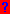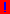# The Matrix Reference Manual

Permission is granted to copy, distribute and/or modify this document under the terms of the GNU Free Documentation License, Version 1.2 or any later version published by the Free Software Foundation; with no Invariant Sections, no Front-Cover Texts, and no Back-Cover Texts.  A copy of the license is included in the section entitled "GNU Free Documentation License".

To cite this manual use: Brookes, M., "The Matrix Reference Manual", [online] http://www.ee.imperial.ac.uk/hp/staff/dmb/matrix/intro.html, 2020. Please see the site accessibility statement here.

## Introduction

This manual contains reference information about linear algebra and the properties of real and complex matrices. The manual is divided into the following sections:

• Main Index: Alphabetical index of all entries.
• Properties : Properties and numbers associated with a matrix such as determinant, rank, inverse, …
• Eigenvalues : Theorems and matrix properties relating to eigenvalues and eigenvectors.
• Special : Properties of matrices that have a special form or structure such as diagonal, traingular, toeplitz, …
• Relations : Relations between matrices such as equivalence, congruence, …
• Decompositions : Decomposing matrices as sums or products of simpler forms.
• Identities : Useful equations relating matrices.
• Equations : Solutions of matrix equations
• Calculus : Differentiating expressions involving matrices whose elements are functions of an independent variable.
• Stochastic : Statistical properties of vectors and matrices whose elements are random numbers.
• Signals : Properties of observation vectors and covariance matrices from stochastic and deterministic signals.
• Examples: 2#2 : Examples of 2#2 matrixes with graphical illustration of their properties.
• Formal Algebra : Formal definitions of algebraic constructs such as groups, fields, vector spaces, …

## Format of Manual Entries

The general format of each entry is as follows:

1. Definition of the term
2. Outline of why it is important
3. Geometric Interpretation
The geometric interpretation of a matrix property or theorem is generally described for 2 or 3 dimensions. Words prefixed by + should be altered appropriately for other dimensions. Thus the word +area should be replaced by volume for 3-D spaces and by hyper-volume for larger spaces.
4. List of properties and theorems:
• Theorems apply to real matrices or complex matrices of arbitrary shape unless explicitly stated.
• Most but not all theorems are also true for matrices whose elements are from other fields; in particular fields with characteristic 2 cause many properties to fail because x = -x does not imply x = 0.
• Matrix dimensions are assumed to allow the sums and products in a theorem.
• If a theorem explicitly involves inv(A) or division by A, then A is assumed to be non-singular.
• If all matrices, vectors and scalars in a theorem are of a particular form, the theorem is prefaced thus: [Real] or [Complex n#n].
• If some matrices are of a particular form, the theorem is prefaced thus: [A,B:n#n, A:symmetric].
•denotes a hypertext link to a proof.
•denotes a hypertext link to an example.

## Notation

The notation is based on the MATLAB software package; differences are notes below. All vectors are column vectors unless explicitly written as transposed.

• Matrices are represented as bold upper case (A), column vectors as bold lower case (a) and real or complex scalars as italic lower case (a).
• The matrix A[2#3] has 2 rows and 3 columns while the column vector a has 4 elements.
• A matrix can be specified explicitly by listing its elements and using a semicolon to separate each row. Thus [1 2 3; 4 5 6] is a matrix with 2 rows and 3 columns. This notation can be used to compose large matrices from smaller ones: [A B;C D]. Each row must have the same total number of columns and each matrix within a row must have the same number of rows.
• Operators
• Operator Precedence:
• (1)  Superscripts, powers and : suffix
• (2)  scalar and matrix multiplication/division
• (3)  ⊗ (Kroneker product)
• (4)   ÷  (elementwise multiplication/division)
• AB, A ÷ B and An denote element-by-element multiplication, division and raising to a power
• AB = KRON(A,B) is the Kronecker product of A and B. If A is m#n and B is p#q then AB is mp#nq and equals the block matrix [a(1,1)B ... a(1,n)B ; ... ; a(m,1)B ... a(m,n)B].
• AB = DIAG(A,B) is the direct sum of A and B.  If A is m#n and B is p#q then AB is m+p#n+q.
• A: (also written vec(A) ) denotes the large column vector formed by concatenating all the columns of A. If A is m#n, then A: = [a1,1 a2,1am,1 a1,2 a2,2am,n]T.
• The following superscripts are used:
• AC denotes the complex conjugate of A.
• AH denotes the conjugate transpose of A. If A is real then AH = AT.
• AR and AI are the real and imaginary parts of  A = AR + j AI .
• AT denotes the transpose of A.
• A-1, A# and A+ denote respectively the inverse, generalized inverse and pseudoinverse of A.
• A-T=(AT)-1=(A-1)T denotes the inverse of the transpose
• A-H=(AH)-1=(A-1)H denotes the inverse of the conjugate transpose
• For real matrices only, A>B means that each element of A is greater than the corresponding element of B. Similar definitions apply to <, >= and <=.
• |A| and ||A||F denote the determinant and Frobenius norm of A.
• ||a|| denotes the euclidean norm of a
• |a| denotes the absolute value of a
• δi,j , the Kronecker delta function, equals 1 if i=j and equals 0 if ij

### Subscripts

• aij or ai,j denotes the element of matrix A in row i of column j. Row and column indices begin at 1.
• A2:5,6:7 denotes the 4#2 submatrix of A consisting of row 2,3,4,5 and columns 6 and 7.
• aj denotes the j'th column of matrix A.
• AX,Y defines a matrix of the same size as X and Y (which must be the same size). Subscripts are taken from corresponding positions in X and Y. [Different from MATLAB]

### Special Matrices

• The dimensions of the following special matrices are normally deduced from context but are occasionally specified explicitly (e.g. 0[m#n]):
• The matrix or vector 0 consists entirely of zeros.
• The matrix or vector 1 consists entirely of ones.
• The matrix I denotes the square identity matrix with 1's down the main diagonal and 0's elsewhere.
• ei is the ith column of I.
• The matrix J denotes the square exchange matrix with 1's along the main anti-diagonal and 0's elsewhere.
• m:n denotes a column vector of length |1+n-m| whose elements go from m to n in steps of +1 or -1 according to whether m<n or m>n. [Different from MATLAB]

### Functions

Several of the functions listed below have different meanings according to whether their argument is a scalar, vector or matrix. The form of the result is indicated by the function's typeface.

• ABS(A) and abs(a) involves taking the absolute value of each matrix or vector element.
• CHOOSE(n,r) is a matrix with n!/(r! (n-r)!) rows, each a different choice of r numbers out of the numbers 1:n. Each row is listed in ascending order.
• CONJ(A), also written AC, is the complex conjugate of A.
• conv(a[m],b[n])[m+n-1] is the convolution of a and b, i.e. a vector whose i'th element is the sum of a(j)b(i-j+1) where j goes from 1 to i.
• det(A), also written |A|, is the determinant of the square matrix A.
• diag(A) is the vector consisting of the diagonal elements of A.
• DIAG(a) is the diagonal matrix whose diagonal elements are the elements of a.
• DIAG(A,B,C), also written ABC, denotes the matrix [A 0 0; 0 B 0; 0 0 C]
• eig(A) is a vector containing the eigenvalues of A. If A is Hermitian, they are sorted into descending order.
• floor(x) is the most positive integer <= x
• INV(A) or A-1 is the inverse of A.
• KRON(A,B) = AB is the Kronecker product of A and B. If A is m#n and B is p#q then AB is mp#nq and equals the block matrix [a(1,1)B ... a(1,n)B ; ... ; a(m,1)B ... a(m,n)B].
• max(a | "condition") is the maximum of a subject to (an optional) "condition".
• min(a | "condition") is the minimum of a subject to (an optional) "condition".
• PERM(n) is a matrix with n! rows, each a different permutation of the numbers 1:n.
• pet(A) is the permanent of A.
• prod(a) is the product of the elements of a.
• prod() is the vector formed by multiplying together the elements of each row of A. [Different from MATLAB].
• rho(A) is the spectral radius of A.
• rows(A) is the number of rows in the matrix A.
• tr(A) is the trace of A.
• rank(A) is the dimension of the subspace spanned by the columns of A.
• sgn(a) equals +1 or -1 according to the sign of a or 0 if a=0.
• sgn(a) equals +1 or -1 according to the signature of the permutation needed to sort the elements of a into ascending order.
• sgn(A) is a vector giving the permutation signatures of each row of A. Each entry equals +1 or -1.
• SKEW(a) is the 3#3 skew-symmetric matrix [0 -a3 a2; a3 0 -a1; -a2 a1 0] where a is a 3-element vector. The vector cross product is given by a × b = SKEW(a) b = -SKEW(b) a. [See skew-symmetric for more properties of SKEW()].
• sum(a) is the sum of the elements of a.
• sum(A) is the vector formed by summing the rows of A. [Different from MATLAB].
• sum(A) is the scalar formed by summing all the elements of A. [Different from MATLAB].
• TOE(a[m+n-1])[m#n] is the m#n matrix with constant diagonals whose i,jth element is ai-j+n.
• TVEC(m,n) is an orthogonal mn#mn permutation matrix whose i,jth element is 1 if j=1+m(i-1)-(mn-1)floor((i-1)/n) or 0 otherwise [see vectorized transpose].
• vec(A), also written A:, denotes the large column vector formed by concatenating all the columns of A. If A is m#n, then A: = [a1,1 a2,1am,1 a1,2 a2,2am,n]T.

## Acknowledgements

No originality is claimed for any of the material in this reference manual. The following books have in particular been very helpful:

• A Survey of Matrix Theory and Matrix Inequalities by M Marcus & H Minc, Prindle, Weber & Schmidt, 1964 / Dover, 1992 [R.12]
• Matrix Analysis and Topics in Matrix Analysis by R A Horn & C R Johnson, CUP 1990/1994, [R.10, R.11]
• Applied Linear Algebra by B. Noble and J.W.Daniel, Prentice-Hall, 1988 [R.13]
• Finite Dimensional Vector Spaces by P.R.Halmos, D Van Nostrand, 1958 [R.8]
• Generalized Inverses by A.Ben-Israel and T.N.E.Greville, Wiley1974 [R.3]
• Matrix Computations by G.H.Golub & C.F.Van Loan, John Hopkins University Press, 1983 ISBN 0-946536-00-7/05-8 [R.7]
• Matrix Methods in Stability Theory by S.Barnett and C.Storey, Nelson, 1970 [R.2]
• Complex Stochastic Processes: An Introduction to Theory and Application by K. S. Miller, Addison-Wesley, 1974 [R.16]

I would like to thank the following people who have made suggestions or corrections to this website and apologise to anyone whose name I have omitted from this list: Gerard Baron, Mike Fairbank, Carlos Fernandes, Thomas Foregger, John Halleck, Olaf Kaehler, Ben Kennedy, James Ng, Kaare Brandt Petersen, Jacopo Piazzi, Walter Tackett, Martin Zimmermann.

This page is part of The Matrix Reference Manual. Copyright © 1998-2017 Mike Brookes, Imperial College, London, UK. See the file gfl.html for copying instructions. Please send any comments or suggestions to "mike.brookes" at "imperial.ac.uk".
Updated: \$Id: intro.html 11291 2021-01-05 18:26:10Z dmb \$